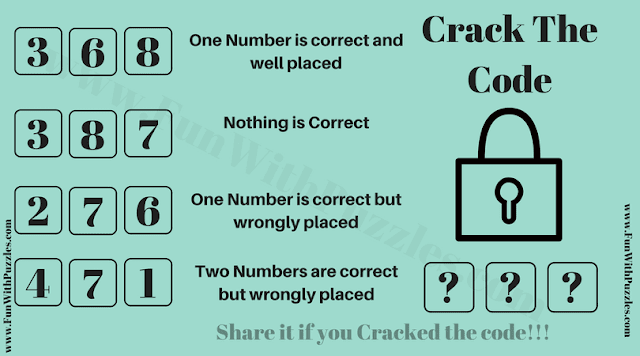## Thursday, January 16, 2020

Once again its time to Crack the Code and open the lock. In this Crack the Code puzzle, you are given 3 digits. Corresponding to these 3 digits, some hints are given regarding correctness to these digits. Your challenge is to decode the correct 3 digit code from these given hints and then open the lock. So are are you ready to crack the code and open the lock?Can you crack the Code and Open the Lock?

The answer to this "Crack the Code Puzzle", can be viewed by clicking on the button. Please do give your best try before looking at the answer.

1.1.yes, you cracked the code :).

2.How do u know that plzz tell

2.3.387 are all wrong

_ 6 _ One number is correct and well placed
_ _ _
2 _ 6
4 _ 1

_ _ _
_ _ _
2 _ _One number is correct but wrongly placed
4 _ 1

Since we already have a correct number for column 2 and row 3 states that it is a right number but in the wrong position then we can put number 2 in column 3

_ _ _
_ _ _
_ _ _
4 _ 1 Two numbers are correct but wrongly placed.  Okay this will debunk my previous answer (_ 6 2) because basically the last row states that both 4 and 1 are the right number and remember that row 3 states that “”One number is correct but wrongly placed. Remember I just blanked out the numbers that we’ve already used but it doesn’t mean that it’s not there. Meaning the statement for row 3 is “Number 6 is correct but wrongly placed” and since number 1’s statement is certain we can just ignore row 3.

_ _ _
_ _ _
_ _ _
4 _ 1 Two numbers are correct but wrongly placed.

Again if they are both in the wrong place and there only spaced left and row 1 is certain for the position of number 6 then what we can do is just flip 4 _ 1 then place it in the remaining columns thus

1.Thanks for explaining the answer to this crack the code puzzle in details.

4.1= 315
2= 325
3= 538
4= 448
Then
6= ?

1.It will be interesting to know the logical reasoning hidden in this puzzle.

5.6.7.8.Yes! I cracked this one too... 164😊

1.9.10.11.